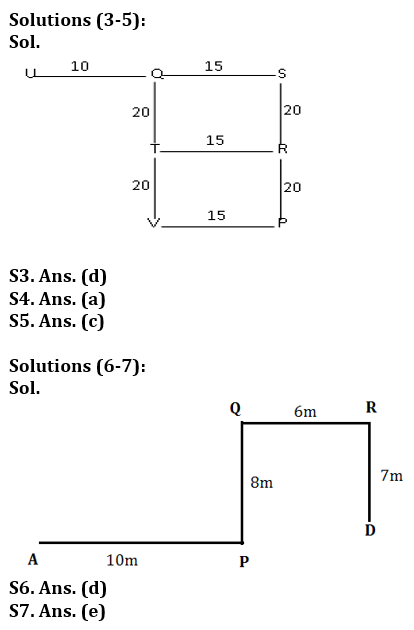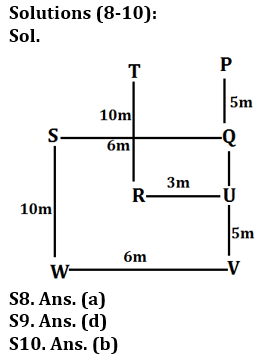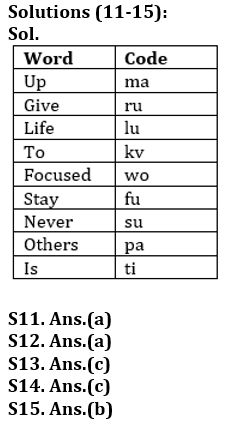Latest Banking jobs   »

# Reasoning Ability Quiz For Bank Foundation 2023-17th March

Q1. A leaves his home and goes straight 20 meters, then turns right and goes 10 meters. He turns left and goes 30 meters and finally turns right and starts walking. If he is moving in the north direction, then in which direction did he start his walking?
(a) East
(b) West
(c) North
(d) South
(e) None of these

Q2. Village B is situated to the north of village A, village C is situated to the east of village B, village D is situated to the left of village A, in which direction is village D situated with respect to village C?
(a) West
(b) South-East
(c) South
(d) North-West
(e) None of these

Directions (3-5): Study the following information carefully and answer the given questions.
Point S is 40 m towards the North of Point P. Point Q is 15 m towards the West of point S. Point U is 25 m towards the West of point S. Point T is 20 m towards the South of point Q. Point R is 15 m towards the East of point T. Point V is 15 m towards the West of point P.

Q3. Which of the following points are in a straight line?
(a) S, T, P
(b) T, V, R
(c) S, Q, V
(d) T, V, Q
(e) U, Q, R

Q4. P is in which direction with respect to R?
(a) South
(b) North
(c) East
(d) West
(e) Cannot be determined

Q5. If a person walks 10 m towards East from point U and then takes a right turn, which of the following points would he reach first?
(a) V
(b) S
(c) T
(d) P
(e) R

Direction (6-7): Study the following information carefully and answer the questions given below:
From point A, Virat walks 10m towards east and reach at point P. Then, he turns towards left and walks 8m to reach point Q. Then, he turns to his right and walks 6m to reach point R. Finally, he turns towards south and walk 7m and reach at point D.

Q6. In which direction is point D with respect to A?
(a) North
(b) East
(c) West
(d) North-east
(e) North-west

Q7. Which among the following is the distance between point P and R?
(a) 6m
(b) 7m
(c) 8m
(d) 9m
(e) 10m

Direction (8-10): Read the information carefully and answer the questions given below:
Point R is 10 m to the south of T. Point U is 3 m to the east of R. Point V is 5 m to the south of U. Point W is 6 m to the west of V. Point S is 10 m to the north of W. Point Q is 6 m to the east of S. Point P is 5 m to the north of Q.

Q8. Which of the following represents the direction of point U with respect to point S?
(a) South-east
(b) North-west
(c) South-west
(d) West
(e) North-east

Q9. How far and in which direction is point T from point P?
(a) 5 metres south
(b) 3 metres east
(c) √34 metres west
(d) 3 metres west
(e) 5 metres north

Q10. What is the shortest distance between point S and point R?
(a) √5m
(b) √34m
(c) 16m
(d) 3√5m
(e) 4m

Direction (11-15): Study the following information carefully and answer the given questions.
In a certain code language,
“Never give up” is coded as “su ru ma”.
“Up is stay” is coded as “fu ti ma”.
“Life give to others” is coded as “pa lu ru kv “.
“Others is to stay focused” is coded as “pa ti kv fu wo”
“Stay others” is coded as “fu pa”.

Q11. How is “up” coded in the given language?
(a) ma
(b) fu
(c) pa
(d) ru
(e) su

Q12. How is “stay” coded in the given language?
(a) fu
(b) ru
(c) su
(d) kv
(e) ti

Q13. How is “Never” coded in the given language?
(a) ru
(b) ma
(c) su
(d) Cannot be determined
(e) None of these

Q14. What can be the code for “focused give to last”?
(a) wo ru kv ti
(b) ru fu kv pa
(c) wo kv ru oq
(d) lu fu ru ti
(e) pa lu fu ma

Q15. Which of the following is coded as “kv”?
(a) life
(b) to
(c) up
(d) focused
(e) give

Solutions

S1. Ans. (b)
S2. Ans. (e)## FAQs

### What is the selection process of the Bank Clerk?

The selection process of the Bank Clerk is Prelims & Mains.

#### Congratulations!Union Budget 2023-24: Free PDF